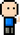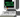# Josh Meets Computer# Feature Ranking - Perturbation Algorithm

April 8, 2018

## Determining Feature Importance

Are there certain features that play a larger role in the final prediction? One way to see the importance of certain features is to corrupt one of the features and see how it affects the prediction capabilities of the model. Features can be ranked based on the results of the model’s prediction capabilities when the feature is rendered useless.

``````import pandas as pd
def rankFeatures(x, y, model, column_names, metric='mean_squared_error'):
"""
Determines which feature contributes most to a prediction
by shuffling each feature before running an evaluation, then
seeing which features cause the greatest impact to the models
prediction capabilities. The act of shuffling has the effect
of rendering the values of one feature useless.
"""
num_features = x.shape
errors = []

base_err = model.evaluate(x, y[:, None])[metric]

for i in range(num_features):
hold = x[:, i]
np.random.shuffle(x[:, i])

shuffled_acc = model.evaluate(x, y[:, None])[metric]
errors.append(shuffled_acc)

x[:,i] = hold

max_error = np.max(errors)
feat_rank = [err / max_error for err in errors]

errors = [round(err - base_err,2)*1000 for err in errors]
data = pd.DataFrame({'Features':column_names, 'Increased Error (\$)':errors, 'Importance':feat_rank})
data.sort_values(by=['Importance'], ascending=, inplace=True)
data.reset_index(inplace=True, drop=True)

return data``````